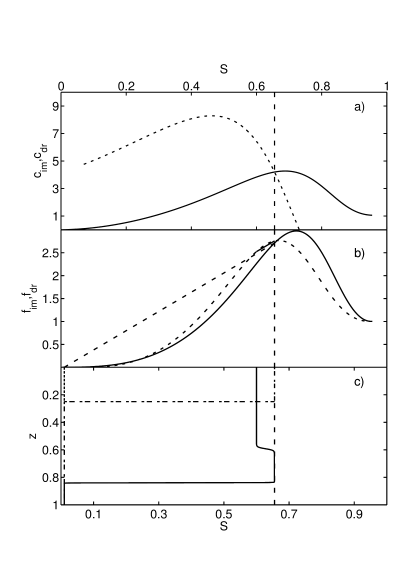Sie sind hier: ICP » R. Hilfer » Publikationen

# 4 Approximate Analytical Solution

## 4.1 Step Function Approximation

[2330.2.1] The basic idea of the analysis below is to approximate the travelling wave profile for long times t with piecewise constant functions (step functions). [2330.2.2] For large [page 2331, §0]    Ca (i.e. in the Buckley-Leverett limit) one may view this approximate profile as a superposition of two Buckley-Leverett shock fronts. [2331.0.1] This is possible by virtue of the fact, that the Heaviside step function

 Θ⁢y=1⁢    ,y>0    or    z/t>c*0⁢    ,y≤0    or    z/t≤c* (35)

can also be regarded as a function of the similarity variable z/t of the Buckley-Leverett problem.

[2331.1.1] In the crudest approximation one can split the total profile for sufficiently large t into the sum

 s⁢y=sim⁢y+sdr⁢y (36)

of an imbibition front

 sim⁢y=Sout+Sin-Sout⁢1-Θ⁢y-z* (36)

located at zim=c*t+z* and a drainage front profile located at zdr=c*t

 sdr⁢y=S*-Sin⁢Θ⁢y⁢1-Θ⁢y-z* (36)

both moving with the same speed c*, where Sin=s- resp. Sout=s are the upper (inlet) resp. lower (outlet) saturations and S* is the maximum (overshoot) saturation. [2331.1.2] The quantity z*=zim-zdr is the distance by which the imbibition front precedes the drainage front, i.e. the width of the tip (=overshoot) region.

## 4.2 Travelling wave solutions

[2331.2.1] The two equations (28) become coupled, if eq.  (20) holds true, because then there is only a single wave speed c* for both fronts. [2331.2.2] At the imbibition discontinuity the Rankine-Hugoniot condition demands

 c*=fim⁢S*-fim⁢SoutS*-Sout:=cim⁢S* (37)

and the second equality (with colon) defines the function cimS. [2331.2.3] Similarly

 c*=fdr⁢S*-fdr⁢SinS*-Sin:=cdr⁢S* (37)

defines the drainage front velocity as a function cdrS of the overshoot S*. Examples of the velocities ci used in the compuations are shown in Figure 1a. [2331.2.4] For a travelling wave both fronts move with the same velocity so that the mathematical problem is to find a solution S* of the equation

 cim⁢S*=cdr⁢S* (38)

obtained from equating eqs. (37b) and (37a) (See Fig. 1a). [2331.2.5] The wave velocity c* is then obtained as cimS* or equivalently as cdrS*.Figure 1: a) Graphical solution of eq.  (38). The dashed curve shows cdrS, the solid line shows cimS. b) Fractional flow functions for drainage (dashed) and imbibition (solid). The two secants (solid/dashed) show the graphical construction of the travelling wave solution with cim=cdr=c*. For better visibility, their line styles have been reversed. Their slopes are equal to each other. c) Numerical solution of eq. (29) using OpenFOAM for the travelling wave constructed graphically in subfigure b). The solution is displayed at time t=0.053222. The monotone initial profile at t=0 is shown as a dash-dotted step.

[page 2332, §1]

## 4.3 General overshoot solutions with two wave speeds

[2332.1.1] Equation (38) provides a necessary condition for the existence of a travelling wave solution of the form of eq.  (36) with velocity c* and overshoot S*. [2332.1.2] More generally, if the saturation plateau SP is larger or smaller than S*, one expects to find non-monotone profiles that are, however, not travelling waves. [2332.1.3] Instead the drainage and imbibition fronts are expected to have different velocities. [2332.1.4] The fractional flow functions with relative permeabilities from eqs. (31) and the parameters from Table 1 give for SP<S* the result

 cim⁢SP

while for SP>S* one has

 cim⁢SP>cdr⁢SP. (40)

[2332.1.5] In this case, for plateau saturations SP<S*, the leading (imbibition) front has a smaller velocity than the trailing (drainage) front. [2332.1.6] Thus the trailing front catches up and the profile approaches a single front at long times. [2332.1.7] For plateau saturations SP>S* on the other hand the trailing drainage front moves slower than the leading imbibition front. [2332.1.8] In this case a non-monotone profile persists indefinitely, albeit with a plateau (tip) width that increases linearly with time.

Parameter Symbol Value Units
system size L 1.0 m
porosity ϕ 0.38
permeability k 210-10 m2
density W ϱW 1000 kg/m3
density O ϱO 800 kg/m3
viscosity W μW 0.001 Pas
viscosity O μO 0.0003 Pas
imbibition exp. αim=βim 0.85
drainage exp. αdr=βdr 0.98
end pnt. rel.p. KWeim 0.35
end pnt. rel.p. KOeim 1
end pnt. rel.p. KWedr 0.35
end pnt. rel.p. KOedr 0.75
imb. cap. press. Pbim 55.55 Pa
dr. cap. press. Pbdr 100 Pa
end pnt. sat. SWiim 0
end pnt. sat. SWidr 0.07
end pnt. sat. SOrim 0.045
end pnt. sat. SOrdr 0.045
boundary sat. Sout 0.01
boundary sat. Sin 0.60
total flux Q 1.196 10-5 m/s
Table 1: Parameter values, their symbols and units